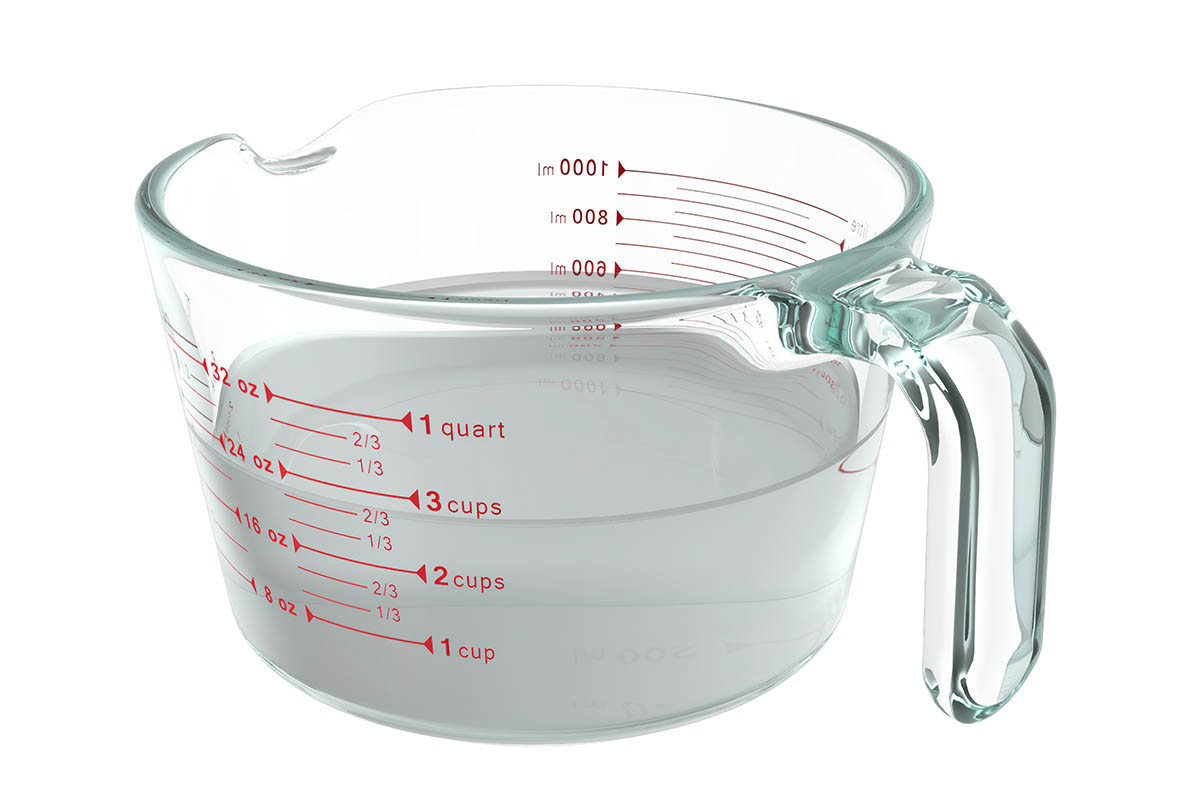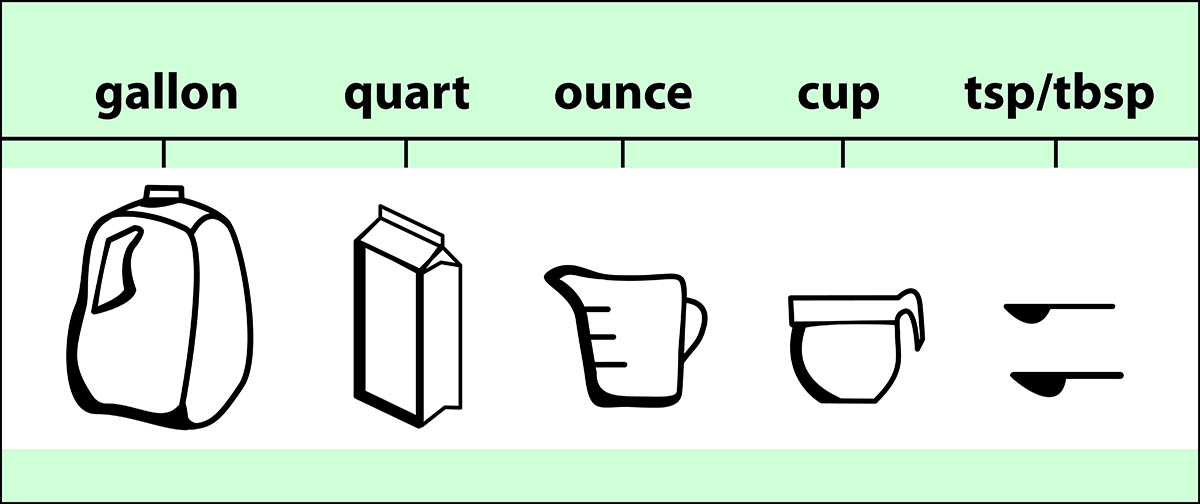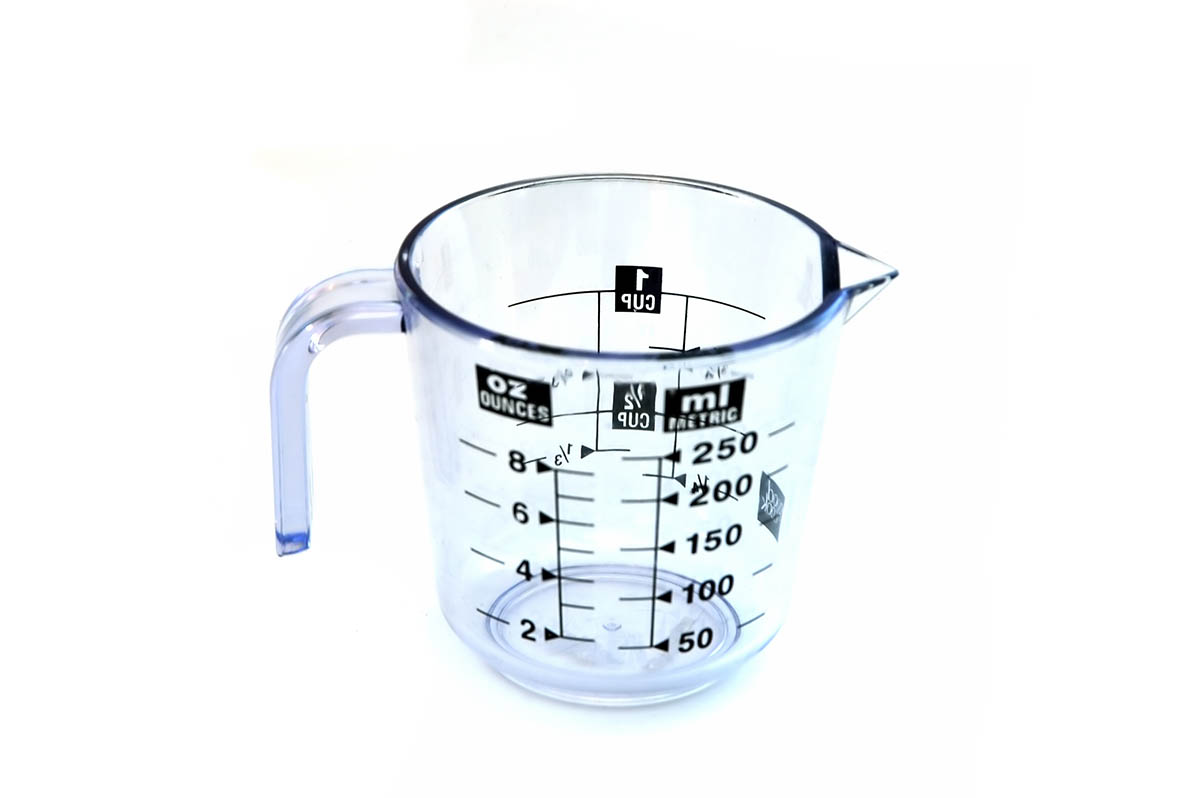# Converting Oz Into Quart

If you are new to cooking or if you are an old hand, you’ll have noticed that recipes across the world use different measuring systems.

The keen eyed among you might have realized that some measurements have the same name but refer to a different unit.It can be very confusing to figure out the number of ingredients you actually need, but today we have compiled an easy to navigate page to help you do the math!

If you need the answer quickly, scroll down to the helpful table at the bottom of the page, otherwise read through this article to really understand the differences!

## What Are The Different Worldwide Measuring Systems?

In the United States of America, we use the Imperial System as our unit of measurement.

We use the term “ounce” to measure either solid items or liquid items, but also use the term “quarts” to do the same thing. I bet you can already see where some of the confusion lies.

In the US we have tried to make this easier, by creating a unit of measurement just for fluids and a different unit of measurement just for dried goods, but for some reason, we decided to use the same names to do this.

Again another layer to the confusion, as some parts of the country used the mixed terms and some use the separated terms, but all use the same name.

Are you confused? Don’t worry most of us are.

The way we differentiate between the two different versions of ounces and quarts is by labeling them the Imperial System and the U.S System.

Almost all of the other countries in the world use the Metric System. You might think this is an exaggeration, but in 2019 Statista confirmed that only three countries still use imperial measurements.

We can’t force every recipe bookseller to convert to the metric system, so instead, we need to figure out the conversions ourselves!

## What Do the Measuring Units Mean?

In the US we mostly use “fluid ounces” to measure the volume of liquid. Notice that this is a “fluid ounce” and not an “ounce”, as a plain “ounce” measures weight.

The other less common measurements we use are quarts, pints, and gallons. A quart is a quarter of a gallon, hence the name.Most other countries use cups or milliliters to measure liquid volumes. Millierlies are from the metric system and cups are from the imperial system.

With all of the different levels of measurements, it’s not hard to see why most of us get confused. The biggest confusion comes when we want to convert a dry measurement to a liquid measurement.

When we do this we have to make sure that the ingredients have the same volume and mass, so that the only variant is the unit of measurement.

If all of this seems like an information overload, don’t worry, I’m going to break it down for you and give you some real answers that you can use in your cooking.

## How to Convert Fluid Ounces into Fluid Quarts (U.S system)?

Rounding up what we already know, a fluid ounce measures the volume of liquids like water and milk.

An easy conversion is to compare fluid ounces to milliliters.

1 fluid ounce (Imperial System) is the same as 28.4 milliliters.

If you are using the U.S Imperial system, 1 fluid ounce is the same as 29.6 milliliters.

The difference between the two imperial systems is tiny right now, but the more ounces you use, the bigger the differences will get. This is why it’s important to know which system you are using.

If you’re not sure, then try to convert all of the ingredients into the same unit of measurement, so the ratio is the same.

Now we know what a fluid ounce’s measurement is, let's have a look at fluid quarts.

• 1 fluid quart (U.S system) is the same as 32 fluid ounces (U.S System).
• 1 fluid quart (Imperial system) is the same as 33.31 fluid ounces (Imperial system).

Knowing that 1 fluid ounce (IS) is 28.4 milliliters means that 1 fluid quart (IS) must be 946 milliliters. And 1 fluid ounce (US) is 29.6 milliliters, meaning that 1 fluid quart (US) must be 947.2 milliliters.

If you have dry foods that you need to convert into liquid ounces, then this is the formula you need to follow:

• 1 dry quart (US) is the same as 37.24 liquid ounces (US)
• 1 dry quart (US) is the same as 38.76 liquid ounces (IS)

### Converting Oz and Quarts into Pints and Cups

We have the fluid quart to fluid ounce conversion, but if you need to convert to different measurements completely, it can get a little tricky.Don’t worry though because we have you covered! The rest of the measurements below are measured in the US system and not the Imperial system (IS).

• 1 fluid quart is the same as 2 pints.
• 1 fluid ounce is the same as 0.0625 pints.
• 1 pint is the same as 16 fluid ounces.
• 1 pint is the same as 0.5 fluid quart.

Now for the cups!

• 1 pint is the same as 2 cups (500 milliliters).
• 1 cup is the same as 0.5 pints.
• 1 cup is the same as 0.125 fluid ounces.
• 4 cups are the same as 32 fluid ounces.
• 4 cups are the same as 1 fluid quart.

### Converting Oz and Quarts into Gallons

Now onto gallons. Quarts is named this way because it measures a quarter of a gallon, so as you have probably guessed this part should be easier.

• 4 quarts are the same as 1 gallon.
• 0.25 gallons is the same as 1 quart.
• 128 ounces is the same as 1 gallon.
• 0.007 gallons is the same as 1 ounce.

### Converting Oz into Quart

Now you know the conversion rates for all the measurements! If you want something to read off quickly, I’ve created a table to help you.

Either read off the table or, if you need a measurement converted that isn’t on the table already, you should be able to figure out the measurement based on the information there.

 Fluid Ounces Quarts Milliliters Cups Pints Gallons 1 0.03125 31.25 0.125 0.0625 0.0078125 4 0.125 125 0.5 0.25 0.03125 8 0.25 250 1 0.5 0.0625 16 0.5 500 2 1 0.125 24 0.75 750 3 1.5 0.1875 32 1 1 000 4 2 0.25 40 1.25 1 250 5 2.5 0.3125 48 1.5 1 500 6 3 0.375 56 1.75 1 750 7 3.5 0.4375 64 2 2 000 8 4 0.5

## Conclusion

Save this page so when you come across a confusing recipe and you need the measurements to be converted, my easy guide can help you quickly get the right amount of ingredients.